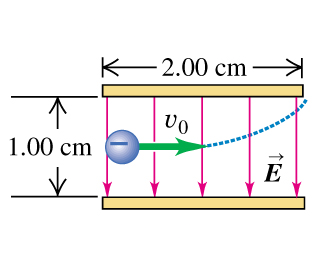# Problem: An electron is projected with an initial speed v0 = 1.10 × 106 m/s into the uniform field between the parallel plates in the figure. Assume that the field between the plates is uniform and directed vertically downward, and that the field outside the plates is zero. The electron enters the field at a point midway between the plates.The electron just misses the upper plate as it emerges from the field. Suppose that in the figure, the electron is replaced by a proton with the same initial speed.Compare the paths traveled by the electron and the proton and explain the differences.

###### FREE Expert Solution

The mass and charge on the particles cause differences in the paths followed.

85% (74 ratings)###### Problem Details

An electron is projected with an initial speed v0 = 1.10 × 106 m/s into the uniform field between the parallel plates in the figure. Assume that the field between the plates is uniform and directed vertically downward, and that the field outside the plates is zero. The electron enters the field at a point midway between the plates.The electron just misses the upper plate as it emerges from the field. Suppose that in the figure, the electron is replaced by a proton with the same initial speed.

Compare the paths traveled by the electron and the proton and explain the differences.